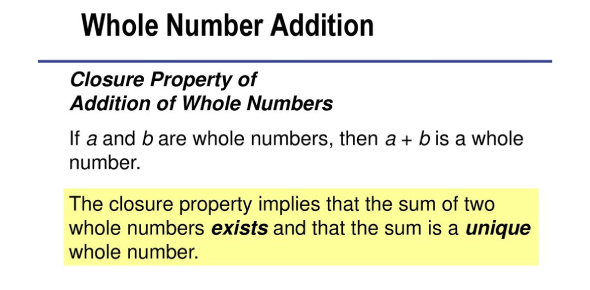# Whole Number Addition Quiz! Trivia

10 Questions | Total Attempts: 135Settings.

• 1.
459 + 731 =
• 2.
587 + 697 =
• 3.
672 + 831 =
• 4.
1567 + 395 =
• 5.
807 + 536 =
• 6.
923 + 522 =
• 7.
6753 + 890 =
• 8.
9807 + 612 =
• 9.
986 + 587 =
• 10.
83 + 158 =
Related Topics